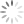Loading, please wait...# Constants in C Programming

Constants

The constants in C are applicable to the values, which do not change during the execution of a program. There are several types of constants in C programming.

### 1) Numerical Constants

a) Integer Constants:

These constants are represented with whole numbers. They require a minimum of 2 bytes and a maximum of 4 bytes of memory.

The following concepts are essential to follow the numerical constants:

1. Numerical constants are represented with numbers. At least one digit is needed for representing the number.

2. The decimal point, fractional part, or symbols are not permitted. Neither blank spaces nor commas as permitted.

3. Integer constant could be either positive or negative or maybe zero.

4. A number without a sign is assumed as positive.

Some valid examples: 10,20,+30,-15,etc.

Some invalid integer constants: 2.3,.235,\$76,3*^6,etc.

Besides representing the integers in decimal, they can also be represented in octal or hexadecimal number system based on the requirement.

Octal number system has base 8 and the hexadecimal number system has base 16. Octal numbers are 0,1,2,3,4,5,6, and 7 and the hexadecimal numbers are 0,1,2,3,4,5,6,7,8,9,A,B,C,D,E and F.

The representation of octal numbers in C would be done with leading digit 0 and for hex representation, with leading OX or Ox.

Examples of octal and hexadecimal numbers:

octal numbers-027,037,072

b) Real Constants:

Real constants are often known as floating-point constants. Real constants can be represented in exponential or fractional form. Integer constants are unfit to represent many quantities. Many parameters or quantities are defined not only in integers but also in real numbers. For example, length, height, price, distance, etc. Also measured in real numbers.

The following concepts are essential to follow the real numbers:

1. The decimal point is permitted.

2. Neither blank spaces nor commas are permitted.

3. Real numbers could be either positive or negative.

4. The number without a sign is assumed as positive.

Examples of real numbers are 2.5, 5.525, 3.14, etc.

The real constants can be written in exponential notation, which contains fractional and exponential parts. For example, the value 2456.123 can be written as 2.4561 X e+3.

### 2) Character Constant

a) Single Character Constants:

A character constant is a single character. It can also be represented with single-digit or a single special symbol or white space enclosed within a pair of single quote marks or character constants are enclosed within single quotation marks.

Example:

a’ , ‘7’, ‘-’

length of a character, at the most, is one character.

Character constants have integer values known as ASCII values. For example, the statement printf (“%c” %d, 65, ‘B’) will display character ‘A’ and 66.

b) String Constants:

A string constant is a sequence of characters enclosed within double quote marks. The string may be a combination of all kinds of symbols.

Example:

“hello” , “India” , “333”, “a”.

There is a programming example for various constants.

* WAP a program on various constants.

``````Void main()
{
int x;
float y;
char z;
double;
clrscr();
x=20;
y=2el;
z=’a’;
p=3. 2E20;
printf(“\n%d %10.2f %c %.21f”,x,y,z,p);
getch();
}``````

OUTPUT:

``20 20.00 a 3200000000000000000000000.00``